# Know Your Numbers: Number Worms

In this worksheet, students find the next number in a sequence given a simple rule.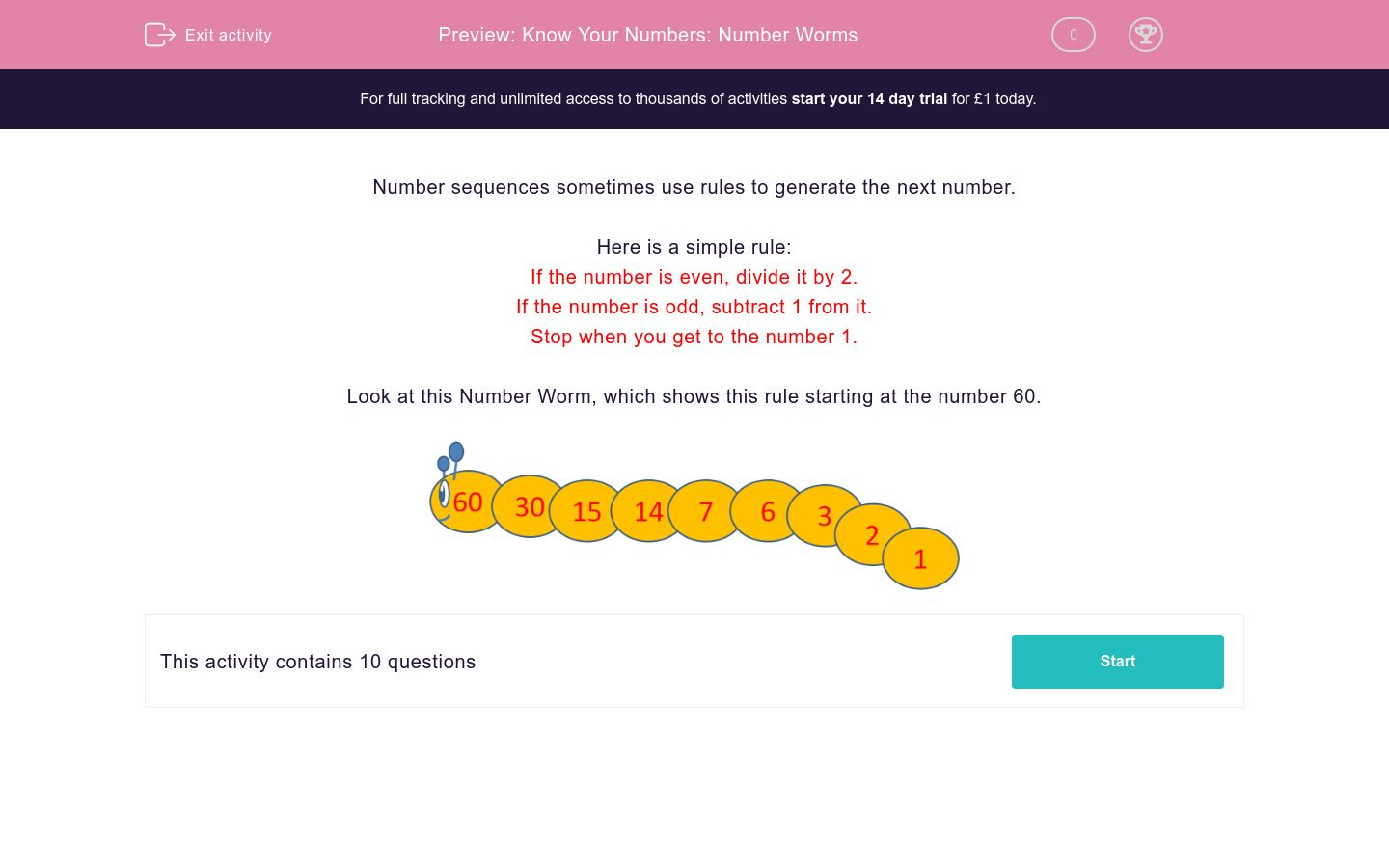Key stage:  KS 2

Curriculum topic:  Maths and Numerical Reasoning

Curriculum subtopic:  Sequences

Difficulty level:### QUESTION 1 of 10

Number sequences sometimes use rules to generate the next number.

Here is a simple rule:

If the number is even, divide it by 2.

If the number is odd, subtract 1 from it.

Stop when you get to the number 1.

Look at this Number Worm, which shows this rule starting at the number 60.If the number is even, divide it by 2.

If the number is odd, subtract 1 from it.

Stop when you get to the number 1.

Work out the values of A, B and C in this number worm, using the rule above.## Column B

A
2
B
5
C
10

If the number is even, divide it by 2.

If the number is odd, subtract 1 from it.

Stop when you get to the number 1.

Work out the values of A, B and C in this number worm, using the rule above.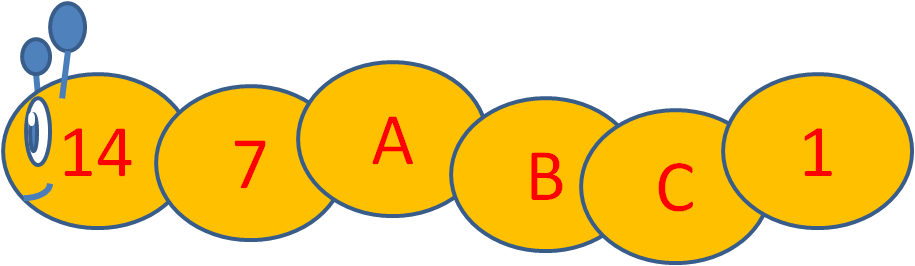## Column B

A
6
B
2
C
3

If the number is even, divide it by 2.

If the number is odd, subtract 1 from it.

Stop when you get to the number 1.

Work out the values of A, B and C in this number worm, using the rule above.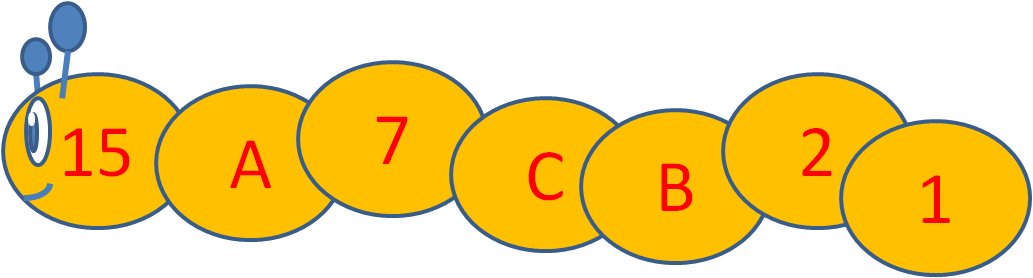## Column B

A
6
B
3
C
14

How many numbers will a Number Worm have starting at the number 6 ?

How many numbers will a Number Worm have starting at the number 16 ?

Will a Number Worm ever have two even numbers together?

Yes

No

Will a Number Worm ever have two odd numbers together?

Yes

No

Look at this Number Worm which follows the same rule as before.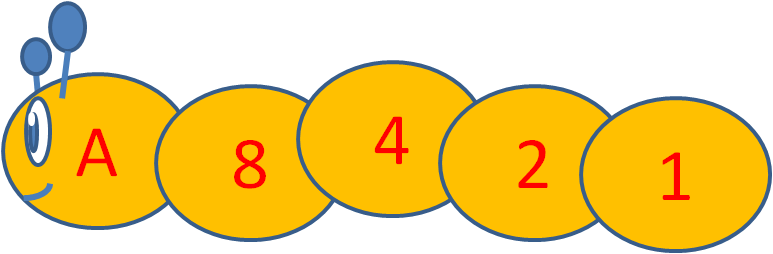Tick the values that A could be.

9

11

12

14

16

Look at this Number Worm which follows the same rule as before.Tick the values that A could be.

30

25

21

60

40

Look at this Number Worm which follows the same rule as before.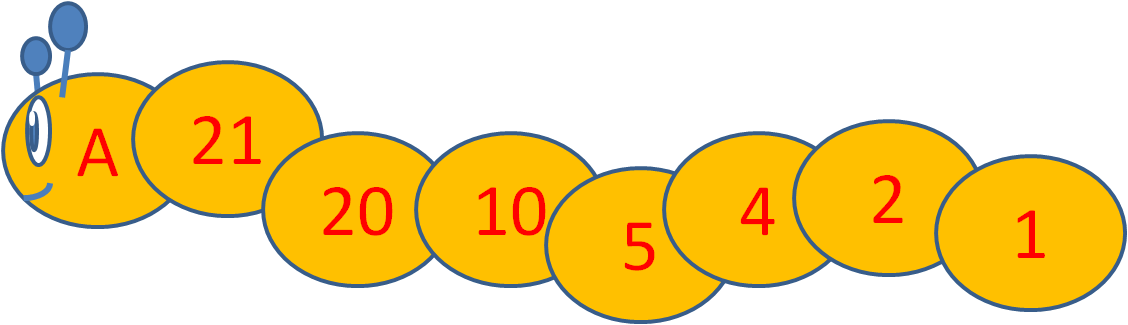Tick the values that A could be.

22

25

21

60

42

• Question 1

If the number is even, divide it by 2.

If the number is odd, subtract 1 from it.

Stop when you get to the number 1.

Work out the values of A, B and C in this number worm, using the rule above.## Column B

A
10
B
5
C
2
• Question 2

If the number is even, divide it by 2.

If the number is odd, subtract 1 from it.

Stop when you get to the number 1.

Work out the values of A, B and C in this number worm, using the rule above.## Column B

A
6
B
3
C
2
• Question 3

If the number is even, divide it by 2.

If the number is odd, subtract 1 from it.

Stop when you get to the number 1.

Work out the values of A, B and C in this number worm, using the rule above.## Column B

A
14
B
3
C
6
• Question 4

How many numbers will a Number Worm have starting at the number 6 ?

4
EDDIE SAYS
6, 3, 2, 1
• Question 5

How many numbers will a Number Worm have starting at the number 16 ?

5
EDDIE SAYS
16, 8, 4, 2, 1
• Question 6

Will a Number Worm ever have two even numbers together?

Yes
• Question 7

Will a Number Worm ever have two odd numbers together?

No
• Question 8

Look at this Number Worm which follows the same rule as before.Tick the values that A could be.

9
16
• Question 9

Look at this Number Worm which follows the same rule as before.Tick the values that A could be.

21
40
• Question 10

Look at this Number Worm which follows the same rule as before.Tick the values that A could be.

42
---- OR ----

Sign up for a £1 trial so you can track and measure your child's progress on this activity.

### What is EdPlace?

We're your National Curriculum aligned online education content provider helping each child succeed in English, maths and science from year 1 to GCSE. With an EdPlace account you’ll be able to track and measure progress, helping each child achieve their best. We build confidence and attainment by personalising each child’s learning at a level that suits them.

Get startedStart your £1 trial today.
Subscribe from £10/month.